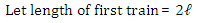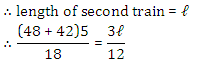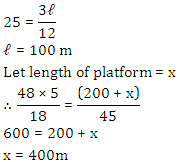Courses

# Quantitative MCQ - 24

## 30 Questions MCQ Test Quantitative Aptitude for Competitive Examinations | Quantitative MCQ - 24

Description
This mock test of Quantitative MCQ - 24 for Quant helps you for every Quant entrance exam. This contains 30 Multiple Choice Questions for Quant Quantitative MCQ - 24 (mcq) to study with solutions a complete question bank. The solved questions answers in this Quantitative MCQ - 24 quiz give you a good mix of easy questions and tough questions. Quant students definitely take this Quantitative MCQ - 24 exercise for a better result in the exam. You can find other Quantitative MCQ - 24 extra questions, long questions & short questions for Quant on EduRev as well by searching above.
QUESTION: 1

### Directions (1-5): In the following questions, two equations numbered I and II are given. You have to solve both the equations and give answer\ I. √x-√6/√x=0 II. y³-6^((3⁄2) )=0

Solution: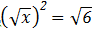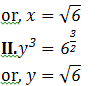QUESTION: 2

### Directions (1-5): In the following questions, two equations numbered I and II are given. You have to solve both the equations and give answer\  I. 3x-2y=10 II. 5x-6y=6

Solution: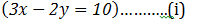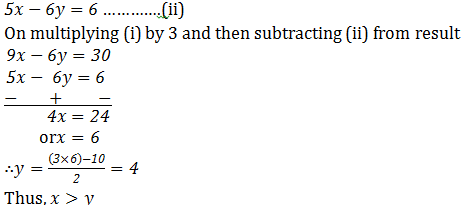QUESTION: 3

### Directions (1-5): In the following questions, two equations numbered I and II are given. You have to solve both the equations and give answer I. x²+x-12=0 II. y²-5y+6=0

Solution: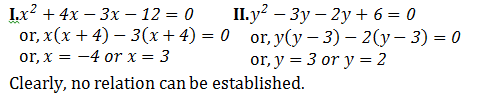QUESTION: 4

Directions (1-5): In the following questions, two equations numbered I and II are given. You have to solve both the equations and give answer\

I. x²+9x+18=0
II. y²-13y+40=0

Solution: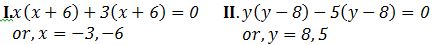QUESTION: 5

Directions (1-5): In the following questions, two equations numbered I and II are given. You have to solve both the equations and give answer

I. √(x+6)=√121-√36
II. y²+112=473

Solution: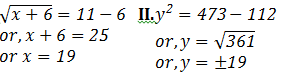QUESTION: 6

Directions (6-10) : What should come in place of the question mark (?) in the following number series.
325, 314, 292, 259, 215, ?

Solution:

The Pattern of the number series is :
325 – 1 × 11 = 314
314 – 2 × 11 = 292
292 – 3 × 11 = 259
259 – 4 × 11 = 215
215 – 5 × 11 = 160

QUESTION: 7

Directions (6-10) : What should come in place of the question mark (?) in the following number series.
45, 46, 70, 141,  ?, 1061.5

Solution:

Series is ×1+1,×1.5+1,×2+1,×2.5+1,……..

QUESTION: 8

Directions (6-10) : What should come in place of the question mark (?) in the following number series.
620,632, 608, 644, 596, ?

Solution:

Series is +(1×12),-(2×12),+(3×12),-(4×12),+(5×12),…….

QUESTION: 9

Directions (6-10) : What should come in place of the question mark (?) in the following number series.
15, 25, 40, 65, ?, 195

Solution:

Series is ×2-(1×5),×2-(2×5),×2-(3×5),×2-(4×5),……

QUESTION: 10

Directions (6-10) : What should come in place of the question mark (?) in the following number series.
120, 320, ?, 2070, 5195, 13007.5

Solution:

Series is ×2.5+20,×2.5+20,×2.5+20,…….

QUESTION: 11

A batsman has certain average of runs is 11 innings. In 12th innings he made 90 runs and thus the average is decreased by 5. His average after 12th inning is

Solution: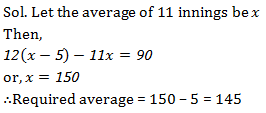QUESTION: 12

A seller labels the marked price of his goods 30% above the cost price but allows a discount of 15% on cash payment. What will be his percentage profit in cash deals?

Solution: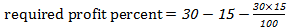= 10.5

QUESTION: 13

Pankaj bought a new house. 2% of the total price of the house will be given to broker as commission. 5% of the price of house is Rs. 2.5 lacs then what is the amount earned by the broker?

Solution: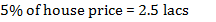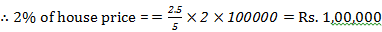QUESTION: 14

The difference between compound interest and simple interest on a certain sum at 10% per annum for 3 years is Rs. 124. What is the sum?

Solution: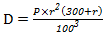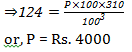QUESTION: 15

Direction (15-19): The following pie charts show the distribution of students of class 10th and 12th in seven different schools namely P, Q, R, S, T, U and V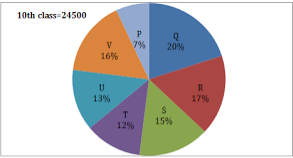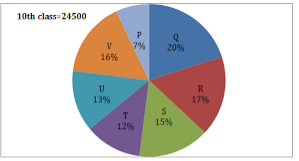Student studying in class 10th in school U are more than the students studying in class 12th of school R by what number?

Solution: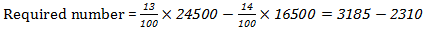= 875

QUESTION: 16

Direction (15-19): The following pie charts show the distribution of students of class 10th and 12th in seven different schools namely P, Q, R, S, T, U and VWhat is the difference between total number of students studying in class 10th in school R, S, T, & U together and students studying in class 12th in school P, Q, and V together?

Solution: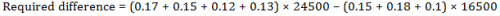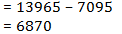QUESTION: 17

Direction (15-19): The following pie charts show the distribution of students of class 10th and 12th in seven different schools namely P, Q, R, S, T, U and V42% of students studying in class 10th in school Q failed in an examination. If the ratio of boys and girls in the failed group is 4 : 3, then find the number of girls who failed in the examination.

Solution:

Required number of girls who failed the examination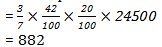QUESTION: 18

Direction (15-19): The following pie charts show the distribution of students of class 10th and 12th in seven different schools namely P, Q, R, S, T, U and VThe average number of students studying in class 12th of school S & T together are approximately what percent more than the number of students studying in class 10th of school P?

Solution: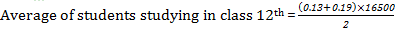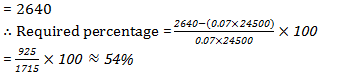QUESTION: 19

Direction (15-19): The following pie charts show the distribution of students of class 10th and 12th in seven different schools namely P, Q, R, S, T, U and VIn a fest, it was decided to select 30% of students studying in class 10th& 20% of students studying in class 12th from every school. What is the average number of students selected for the fest?

Solution: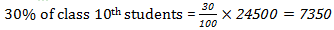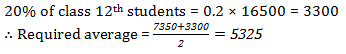QUESTION: 20

In a class of 240 students, each student got sweets that are 15% of the total number of students. How many sweets were there?

Solution:

No. of student = 240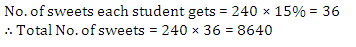QUESTION: 21

Seema purchased an item for Rs 9,600 and solid it for a loss of 5 percent. From that money, she purchased another item and sold it for a gain of 5 percent. What was her overall gain/loss?

Solution: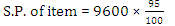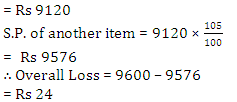QUESTION: 22

The compound interest (in Rupees) on Rs 5,600 for 3/2 years at 10% per annum, compounded annually is

Solution: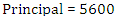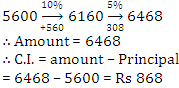QUESTION: 23

B is 3 years older than A and B is also 3 years younger than ‘C’. 3 years hence, the respective ratio between the ages of A and C will be 4: 5. What is the sum of the present ages of A, B and C?

Solution:

Let the present age of A = x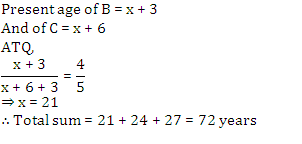QUESTION: 24

The average age of 80 boys in a class is 15 years. The average age of a group of 15 boys in the class is 16 years and the average age of another 25 boys in the class is 14 years. What is the average age of the remaining boys in the class?

Solution: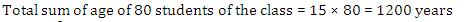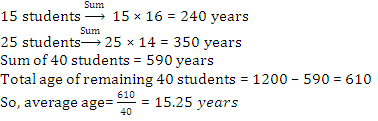QUESTION: 25

Directions (25-29): What should come in place of question mark (?) in the following number series?

2, 13, 67, ?, 817, 1639

Solution: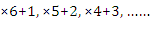QUESTION: 26

Directions (25-29): What should come in place of question mark (?) in the following number series?

1, 2, 6, 21, ?, 445, 2676

Solution: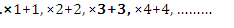QUESTION: 27

Directions (25-29): What should come in place of question mark (?) in the following number series?

8, 52, ?, 1287, 4504.5, 11261.25, 16891.875

Solution: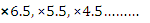QUESTION: 28

Directions (25-29): What should come in place of question mark (?) in the following number series?

250000, 62500, 12500, 3125, 625, ?, 31.25

Solution: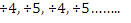QUESTION: 29

Directions (25-29): What should come in place of question mark (?) in the following number series?

588, 563, 540, 519, ?, 483, 468

Solution: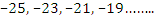QUESTION: 30

A train travelling at 48 km/hr crosses another train, having half its length and travelling in opposite direction at 42 km/hr in 12 seconds. It also passed a railway platform in 45 seconds. The length of the rail platform is

Solution: